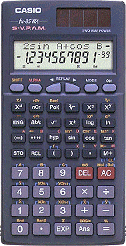Search IntMath
Close

# 4. Polar Form of a Complex Number

by M. Bourne

We can think of complex numbers as vectors, as in our earlier example. [See more on Vectors in 2-Dimensions].

We have met a similar concept to "polar form" before, in Polar Coordinates, part of the analytical geometry section.

### Why Polar Form?

In the Basic Operations section, we saw how to add, subtract, multiply and divide complex numbers from scratch.

However, it's normally much easier to multiply and divide complex numbers if they are in polar form.

Our aim in this section is to write complex numbers in terms of a distance from the origin and a direction (or angle) from the positive horizontal axis.

We find the real (horizontal) and imaginary (vertical) components in terms of r (the length of the vector) and θ (the angle made with the real axis):

From Pythagoras, we have: r^2=x^2+y^2 and basic trigonometry gives us:

tan\ theta=y/xx=r\ cos theta y = r\ sin theta

Multiplying the last expression throughout by j gives us:

yj = jr\ sin θ

So we can write the polar form of a complex number as:

x + yj = r(cos θ + j\ sin θ)

r is the absolute value (or modulus) of the complex number

θ is the argument of the complex number.

There are two other ways of writing the polar form of a complex number:

r\ "cis"\ θ [This is just a shorthand for r(cos θ + j\ sin θ)]

r\ ∠\ θ [means once again, r(cos θ + j\ sin θ)]

NOTE: When writing a complex number in polar form, the angle θ can be in DEGREES or RADIANS.

### Example 1

Find the polar form and represent graphically the complex number 7 - 5j.

We need to find r and θ.

r=sqrt(x^2+y^2)

= sqrt(7^2 +(-5)^2)

= sqrt(49+25)

= sqrt(74) ~~8.6

To find θ, we first find the acute angle α (see Trigonometric Functions of Any Angle if you are rusty on this):

alpha = tan^(-1)(y/x)

= tan^(-1)(5/7)

~~35.54^text(o)

Now, 7 - 5j is in the fourth quadrant, so

θ = 360^@ - 35.54^@ = 324.46^@

So, expressing 7 - 5j in polar form, we have:

7 - 5j  = 8.6 (cos 324.5^@ + j\ sin\ 324.5^@)

We could also write this answer as 7 - 5j = 8.6\ "cis"\ 324.5^@.

Also we could write: 7 - 5j = 8.6 ∠ 324.5^@

The graph is as follows:

### Example 2

Express 3(cos 232^@+ j sin 232^@) in rectangular form.

This is how the complex number looks on an Argand diagram. The distance from the origin is 3 and the angle from the positive R axis is 232^@.

To get the required answer, we simply multiply out the expression:

3(cos 232^@ +j\ sin 232^@) = 3\ cos 232^@ + j (3\ sin 232^@)

= -1.85 - 2.36j

### Exercises

1. Represent 1+jsqrt3 graphically and write it in polar form.

θ = arctan(sqrt(3)/1) = 60^@

r = sqrt((sqrt(3))^2 + 1^2) = sqrt(4) = 2

(We recognise this triangle as our 30-60 triangle from before.)

So

1 + j sqrt 3 = 2\ ∠\ 60^@  = 2(cos 60^@ + j\ sin 60^@)

2. Represent sqrt2 - j sqrt2 graphically and write it in polar form.

r=sqrt(x^2+y^2)

= sqrt( (sqrt(2))^2 +(sqrt(2))^2)

= sqrt(2+2)

= sqrt(4)

 =2

To find θ, we first find the acute angle alpha:

alpha = tan^(-1)(y/x)

=tan^(-1)(sqrt(2)/(sqrt(2)))

 ~~ 45^text(o)

The complex number is in the 4th quadrant, so

θ = 360^@ - 45^@ = 315^@

So we can write:

sqrt2 - jsqrt2 = 2\ ∠\ 315^@

 = 2(cos315^@ + jsin315^@)

3. Represent graphically and give the rectangular form of 6(cos 180^@+ j\ sin 180^@).

We can read the rectangular form of this number from the graph.

6(cos 180^@ + j\ sin 180^@) = -6

4. Represent graphically and give the rectangular form of 7.32 ∠ -270°

Once again, a quick look at the graph tells us the rectangular form of this complex number.

7.32\ ∠ -270^@= 7.32j

## And the good news is...Now that you know what it all means, you can use your calculator directly to convert from rectangular to polar forms and in the other direction, too.

### Online Polar Calculator

Also, don't miss this interactive polar converter graph, which converts from polar to rectangular forms and vice-versa, and helps you to understand this concept:

Online polar to rectangular calculator

## Problem SolverThis tool combines the power of mathematical computation engine that excels at solving mathematical formulas with the power of GPT large language models to parse and generate natural language. This creates math problem solver thats more accurate than ChatGPT, more flexible than a calculator, and faster answers than a human tutor. Learn More.